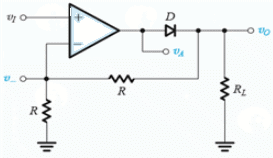Test: Rectifiers

# Test: Rectifiers

Test Description

## 10 Questions MCQ Test Analog Electronics | Test: Rectifiers

Test: Rectifiers for Electrical Engineering (EE) 2023 is part of Analog Electronics preparation. The Test: Rectifiers questions and answers have been prepared according to the Electrical Engineering (EE) exam syllabus.The Test: Rectifiers MCQs are made for Electrical Engineering (EE) 2023 Exam. Find important definitions, questions, notes, meanings, examples, exercises, MCQs and online tests for Test: Rectifiers below.
Solutions of Test: Rectifiers questions in English are available as part of our Analog Electronics for Electrical Engineering (EE) & Test: Rectifiers solutions in Hindi for Analog Electronics course. Download more important topics, notes, lectures and mock test series for Electrical Engineering (EE) Exam by signing up for free. Attempt Test: Rectifiers | 10 questions in 10 minutes | Mock test for Electrical Engineering (EE) preparation | Free important questions MCQ to study Analog Electronics for Electrical Engineering (EE) Exam | Download free PDF with solutions
 1 Crore+ students have signed up on EduRev. Have you?
Test: Rectifiers - Question 1

### Which of the following isn’t a type of rectifier?

Detailed Solution for Test: Rectifiers - Question 1

All of the mentioned are different types of a rectifier.

Test: Rectifiers - Question 2

### For a half wave or full wave rectifier the Peak Inverse Voltage of the rectifier is always

Detailed Solution for Test: Rectifiers - Question 2

The peak input voltage is smaller than the input voltage due to the presence of diode(s). A single diode reduces the output voltage by approximately 0.7V.

Test: Rectifiers - Question 3

### For a half-wave rectifier having diode voltage VD and supply input of VI, the diode conducts for π – 2Θ, where Θ is given by

Detailed Solution for Test: Rectifiers - Question 3

The diode doesn’t conducts when VD ≥VI . Hence Θ = sin-1 (D/VI).

Test: Rectifiers - Question 4

Bridge rectifier is an alternative for

Detailed Solution for Test: Rectifiers - Question 4

Bridge rectifier is a better alternative for a full wave rectifier.

Test: Rectifiers - Question 5

Which of the following is true for a bridge rectifier?

Detailed Solution for Test: Rectifiers - Question 5

All of the given statements are true for a bridge rectifier.

Test: Rectifiers - Question 6

The diode rectifier works well enough if the supply voltage is much than greater than 0.7V. For smaller voltage (of few hundreds of millivolt) input which of the following can be used?

Detailed Solution for Test: Rectifiers - Question 6

For the supply voltages less than 0.7V super diodes are used.

Test: Rectifiers - Question 7

A simple diode rectifier has ‘ripples’ in the output wave which makes it unsuitable as a DC source. To overcome this one can use

Detailed Solution for Test: Rectifiers - Question 7

A capacitor is parallel with a resistor can only makes ripples go away. Series connection will become equal to an open circuit once the capacitor is fully charged.

Test: Rectifiers - Question 8

Consider a peak rectifier fed by a 60-Hz sinusoid having a peak value Vp = 100 V. Let the load resistance R = 10 kΩ. Calculate the fraction of the cycle during which the diode is conducting

Detailed Solution for Test: Rectifiers - Question 8

w Δt ~ √(2Vr/Vp)
Θ = √(2 X 2/100)
Θ = 0.2 rad or 3.18% of the cycle

Test: Rectifiers - Question 9

(Q.9-Q.10) The op amp in the precision rectifier circuit is ideal with output saturation levels of ±12 V. Assume that when conducting the diode exhibits a constant voltage drop of 0.7 V.Find V when VI is -1V.

Detailed Solution for Test: Rectifiers - Question 9

VI = -1v
Vo = 1v
VA = 1.7v
V = 0v
Virtual gnd as negative feedback is closed through R.
VI > 0 D1 conducts D2 cutoff.
VI < 0 D2 conducts D1 cutoff.
V0 ⁄ VI = -1.

Test: Rectifiers - Question 10

Find V0 when VI is 2V.

Detailed Solution for Test: Rectifiers - Question 10

: VI = 2v
Vo = 0v
VA = -0.7v
V = 0v
Virtual gnd as negative feedback is closed through R.
VI > 0 D1 conducts D2 cutoff.
VI < 0 D2 conducts D1 cutoff.
V0 ⁄ VI = -1.

## Analog Electronics

11 videos|57 docs|108 tests(Scan QR code)